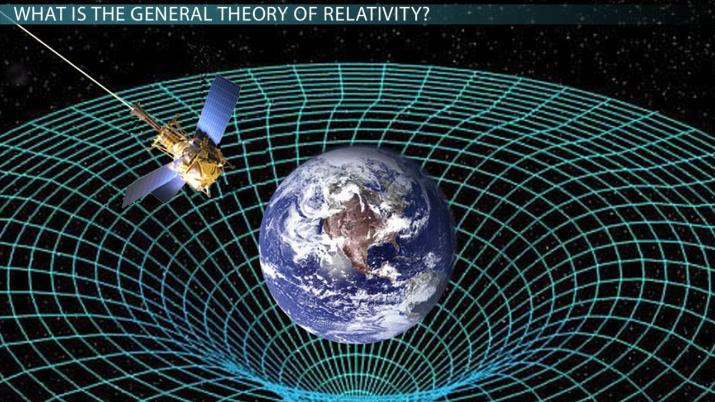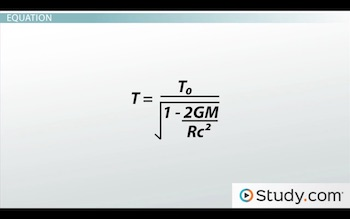# General Theory of Relativity: Analysis & Practice Problems

Lesson Transcript
Instructor: David Wood

David has taught Honors Physics, AP Physics, IB Physics and general science courses. He has a Masters in Education, and a Bachelors in Physics.

Einstein's General Theory of Relativity is founded upon the principles of Newton's laws. Learn some of the formulas, features of inertial and gravitational mass, and analyze them through a practice problem. Updated: 10/31/2021

## What is the General Theory of Relativity?

General relativity is a theory of gravity proposed by Einstein in the early 20th century, which is an expansion on Newton's classical theory of gravity. While Newton's laws work fine on the scale of our everyday lives, it turns out that when you look at really large scales - like the sizes of planets or beams of light traveling many light years, it doesn't quite work.

General relativity is exceedingly complex and has many consequences, so we'll just talk about some of the most important ones. First of all, general relativity says that the gravitational mass for an object is the same as the inertial mass. What does this mean?

Well, inertial mass is the mass you find in the equation F = ma -- it's the mass that makes it harder to accelerate a heavier object. The gravitational mass on the other hand, is the mass you find in gravitational equations, like F = GMm/r^2, and is the mass that gives rise to gravitational forces between objects. Although we tend to assume that these two masses are the same number, that a 1 kilogram mass means we need to put a 1 in place of m in both equations, this wasn't a foregone conclusion. Perhaps these two values of m are only extremely similar, and we just weren't being accurate enough to be sure. Einstein's theory said that they are EXACTLY the same, and later experiments showed this to be true.

One consequence of this is that anything with momentum must have not only an inertial mass, but also a gravitational mass. A physicist, called De Broglie, had shown that even photons of light have a momentum, and so according to general relativity, they must also have a gravitational mass. This surprising result means that photons of light should be affected by gravitational fields, just as regular particles are. So light can be bent by large massive objects. And in fact, the stars we see near to our Sun are not where they appear, because the light has been bent towards our Sun as the light passed by.

Another important consequence of general relativity is that time flows at different rates depending on the strength of the gravitational field. A clock in a larger gravitational field - near to the Sun for example - will tick more slowly than a clock in a weaker field on Earth. The effect is extremely tiny, but this is the reason that such weird stuff happens when people go near to super-massive black holes.An error occurred trying to load this video.

Try refreshing the page, or contact customer support.

Coming up next: Theory of Special Relativity: Definition & Equation

### You're on a roll. Keep up the good work!

Replay
Your next lesson will play in 10 seconds
• 0:02 What is the Theory?
• 2:33 Equation
• 3:44 Practice Problem
• 4:42 Lesson Summary
Save Save

Want to watch this again later?

Timeline
Autoplay
Autoplay
Speed Speed

## EquationThe equation we're going to use for general relativity looks like the one above and is called the gravitational time dilation equation. It says that T equals T-zero, divided by the square root of 1 minus 2 times big G, times M, divided by R-c-squared.

What do all these terms mean? Well, T is the time that passes for a clock OUTSIDE a gravitational field, T-zero is the time that passes for a clock INSIDE the field, big G is the gravitational constant of the universe, M is the mass of the object creating the gravitational field, R is the radius your clock is away from that object, and c is the speed of light. T and T-zero are measured in seconds, G is always 6.67 x 10^-11, M is measured in kilograms, R is in meters, and c is in meters per second.

So all you have to do is plug in some numbers to discover how different the passage of time will be in one location compared to another. There are many other equations in general relativity - more than we could possibly talk about in a single video lesson. But this is a simple one that can help us do some meaningful calculations.

## Practice Problem

Okay, time for a practice problem. A spaceship is orbiting the Sun at a radius of 430,000 meters for 10,000 seconds. If the mass of the Sun is 2 x 10^30 kilograms, how much time passed at another location a long way away from the Sun?

To unlock this lesson you must be a Study.com Member.

## Practice:General Theory of Relativity: Analysis & Practice Problems Quiz

1/5 completed

### In the equation F = ma, what kind of mass is m?

Create Your Account To Take This Quiz

As a member, you'll also get unlimited access to over 84,000 lessons in math, English, science, history, and more. Plus, get practice tests, quizzes, and personalized coaching to help you succeed.

It only takes a few minutes to setup and you can cancel any time.

### Register to view this lesson

Are you a student or a teacher?